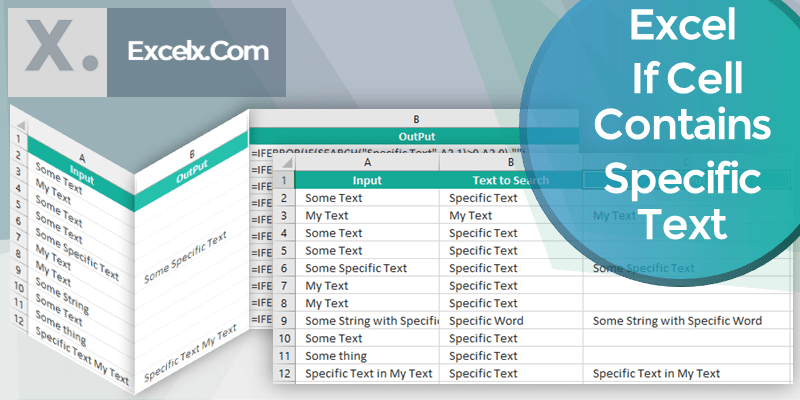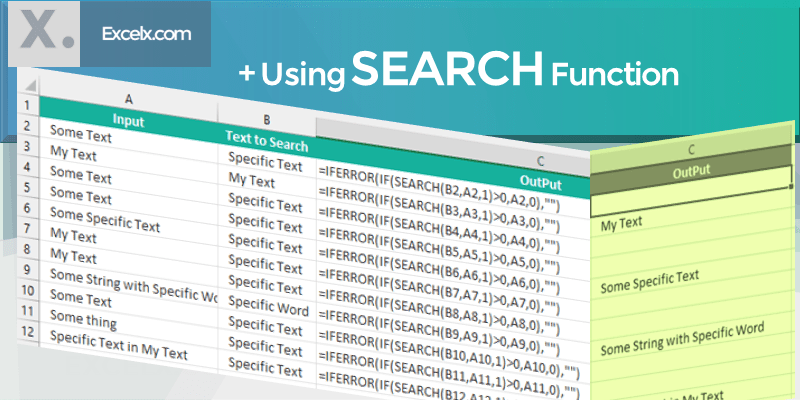Here is the the Excel formula to check if cell contains specific text then return value. Often, we need to search for a text in a cell or range of cells in the Excel, return if it contains a specific text or string. Following is the simple formula to find specific text in given cells and return its values.

## If Cell Contains Specific Text Then Return Value

Following is the Excel formula to find If Cell Contains Specific Text Then Return Value. This example used IF and COUNTIF functions to check for a value in a Cell and return required value.

`=IF(COUNTIF(A1,"*Specific Text*"),"Yes","No")`

You can see that we are counting the cells with specific text and returning the Value ‘Yes’ if found, ‘No’ if not found in the Cell.

### If Cell Contains Specific Text Then Return Value – Using COUNTIF Function

We can return the Cell value if using the same functions. You can also change the Specific Text to a Cell reference if you wants to make the function more dynamic.

`=IF(COUNTIF(A1,"*"&B2&"*"),A1,"")`

the above function returns the Cell Value if a specific text found in the Cell, otherwise it returns Blank.### If Cell Contains Specific Text Then Return Value – Using SEARCH Function

You can also achieve this by using Search Function. Below is the Excel formula to find If Cell Contains Specific Text Then Return Value. In our example, we have input data in Cell A2:A12 and We will Return the Values in Range B2:B12.

Here the Excel formula to Return Value If Cell Contains Specific Text :

`=IFERROR(IF(SEARCH(B2,A2,1)>0,A2,0),"")`

Values Passed in the SEARCH Function

• Parameter 1: B2=”find_text”, the text where you can replace with the specific text to be searched in another text
• Parameter 2: A2=”within_text”, where you will search for ‘find_text’
• Parameter 3: 1=Character number which you want start you search in within_text from the left.### Formula to Determine If Cell Contains Specific Text

Here is the simple formula to check if cell contains specific text or not. You can search for a text and return the required values (for example: Yes, No), if finding text existing in within cell.

`=IF(COUNTIF(A2,"*Specific Text*"),"Yes, it is Found", "Not Found")`

Here COUNTIF function determines if a specific text exist in a Cell. And the IF function return “Yes” if Found.

### Formula to Determine If Cell Contains Certain Text

You can use the following formula to Determine If Cell Contains Certain Text. You can change any text in the Cell B1 and you can see the result instantly.

`=IF(COUNTIF(A1,"*Certain Text*"),"TRUE","FALSE")`

We can make this more dynamic by passing certain text using a cell as shown below:

`=IF(COUNTIF(A1,"*" &B1 &"*"),"TRUE","FALSE")`

### Excel VBA If Cell Contains Certain Text

Here is the Excel VBA to check if Cell contains certain text. You can change the cell reference to any cell and specificText to any text.
``` Sub MacroToCheckIfCellContainsSpecificText_vba()```

``` Set Cell = Cell(1, 4) ' You can change this specificText = "Specific Text" ' You can change this ```

```If UCase(Cell.Value) Like "*" & UCase(specificText) & "*" Then MsgBox "Found" Else MsgBox "Not Found" End If End Sub ```

1. Dhuha April 26, 2022 at 10:51 am - Reply

Thank you very much for this – What if we want to check if the cell contain this text?

• PNRao May 3, 2022 at 2:09 am - Reply

=IF(COUNTIF(A1,”*This Text*”),”Yes”,”No”)

2. Lost in Excel June 15, 2022 at 6:45 am - Reply

If any cell in columns C;F have “x”, then return corresponding value (name) from row 1 to column B, on each row. Return all matches, if found.

If this would be just four columns and four rows, I’d do it manually, but my table has hundreds of rows and more than ten columns to check, so its too time consuming to do it manually.

Formula in Cell B2 [=IF(C2=””;””;”JOHN”)] returns only one result and the formula doesn’t accept more arguments to IF. How to do this?

This is what it should look like.
A B C D E F
1 John Mary Steve Sarah
2 Day 1 John, Mary x x
3 Day 2 Mary, Steve x x
4 Day 3 John, Sarah x x
….
n+1 Day n+1

• PNRao June 20, 2022 at 1:13 pm - Reply

You can use the TEXTJOIN and IF Function to get the corresponding (comma separated) list of the matched string:

`=TEXTJOIN(", ",TRUE,IF(C2:F2="x",\$C\$1:\$F\$1,""))`

This look for “x” in the Column C:F (Relative reference) and Join the all corresponding values from C1:F1 (absolute reference).

3. TJ June 17, 2022 at 12:55 am - Reply

How about start and end strings?

• PNRao June 20, 2022 at 1:00 pm - Reply

To Check if A Cell Starting with Certain String, use the following formula:

`=IF(COUNTIF(A1,"Specific Text*"),"Yes","No")`

To Check if A Cell Ending with Certain String, use the following formula:

`=IF(COUNTIF(A1,"*Specific Text"),"Yes","No")`
4. Fidele Niyongira June 21, 2022 at 11:29 am - Reply

Thank you so much for your help guys you are really amazing.

• PNRao June 21, 2022 at 4:56 pm - Reply

You are Welcome!

5. Linda June 22, 2022 at 6:20 pm - Reply

I have a jumbled up column of data like this:
“2022 LI List”,”2021 CR Leadership”,”#CR”,”2021 IG List”,”Joe CR”
I want to put each item into its own column across the page so we can sort and analyze the data by row, by person the data is assigned to. It is currently a single column separated by comma and quotation marks and not in order. In the end I want each row to have a column for each item filled with that value.

• PNRao July 1, 2022 at 2:57 am - Reply

Please label the columns and provide the details about the example data:
“2022 LI List”,”2021 CR Leadership”,”#CR”,”2021 IG List”,”Joe CR”

We understand that items (1,2 and 4) in your data contains 3 columns, the 3rd one has only one, the last item have two. Please provide more details, how you wants to see in your output.

6. jay June 29, 2022 at 7:46 pm - Reply

Anyone know how I can combine these two statements so it will work? ‘
=IF(1,QUERY(StudentList!\$A:\$B, “Select B where A = “&E3,0),””)
=IF(2,QUERY(StudentList1!\$A:\$B, “Select B where A = “&E3,0),””)
When I put the first IF statement in the cell it works correctly, but when I try to add the 2nd IF statement followed by a comma it gives me an error message. Basically I need this formula to read another cell and depending on if the value in that cell is 1 or 2 I will either need it to go to the StudentList page or the StudentList1 page and grab the data. Thanks.

• PNRao July 1, 2022 at 3:10 am - Reply

``` =IF(1,"Process 1",IF(2,"Process 2",""))```

``` ```

```=IF(1,QUERY(StudentList!\$A:\$B, "Select B where A = "&E3,0),IF(2,QUERY(StudentList1!\$A:\$B, "Select B where A = "&E3,0),"")) ```

7. Pete July 7, 2022 at 3:58 pm - Reply

Hello
I have been trying all afternoon to figure out this equation but am lost. Am drafting an expense form where if a certain department is chosen VAT needs to be worked out.
Have already set up an equation to give me Yes or No is one of the departments is selected, then I need to use the gross sales amount of invoice and find 80% of this.

8. Nikola M August 23, 2022 at 5:00 pm - Reply

Hi, thanks for this tutorial. Now I have another problem – in column C I have a lot of blank spaces, and I want to make a table with data in it only. How do you make this?
i.e. with the CountIf function, in column C now I have (as mentioned above):
Some Text

Some Text
Some Text

Some Text
But I want to have a table with no blank spaces in between:
Some Text
Some Text
Some Text
Some Text

How to do this? Thanks

• PNRao September 11, 2022 at 7:12 am - Reply

Assuming your Data in Column C start from Row 2 (C2):

Step1: Return the Non Blank Index in Column D. Paste the Below Formula at Range D2 and Fill down:

```=IF(C2="","",MAX(D\$1:D1)+1)
```

Step2: Return the Non Empty Data into Column E. Paste the Below Formula at Range E2 and Fill down:

```=IFERROR(INDEX(\$C\$2:\$C\$11,MATCH(ROW()-ROW(\$E\$1),\$D\$2:\$D\$11,0)),"")
```